## Exponent Basics

### Exponential Basic Training

Okay Shmoopers, let's begin by getting the basics out of the way. This is the stuff you might already know. To be sure everyone is on the same page, let's do this thing.

Exponential expressions have two basics parts: a base and an exponent. So if we consider the expression 35, 3 would be the base while 5 is the exponent. In this example, we can think of 3 being multiplied by itself 5 times.

35 = 3 · 3 · 3 · 3 · 3 = 243

For those of you playing at home, this does not mean that you need to multiply 3 times 3 times 3 times 3 times 3 on your calculator every single time you evaluate an exponent. Instead, most calculators have a button that either looks like y x or ^ that you can use to evaluate exponents. This makes life much, much easier. The ^ thing is called a "caret," by the way.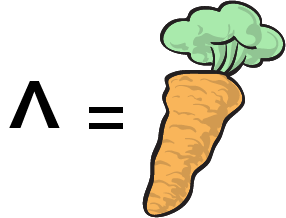Now that you know what these things are, let's simplify 'em. Here are a few rules to get you started:

If you multiply like bases, add the exponents. This is the product rule.

x3 · x4 = x(3 + 4) = x7

Think about this for a second: x3 is really like x · x · x, and x4 is really like x · x · x · x. Multiplied together, we have (x · x · x) · (x · x · x · x). That's 7 x's. So our simplified answer has got to be x7.

If one exponent is raised to another, you multiply the exponents. This is the power rule.

(x3)2 = x(3 · 2) = x6

If you understand the product rule, the power rule really isn't too terrible. In this case, x3 is squared. This means we have two x3's multiplied together: x3 · x3. Using the rule we've already established, we can get our answer.

Two other pieces of info that might interest you are what happens when the exponent is either 1 or 0. For instance, imagine you were asked to simplify x1. Luckily, this is just x. This is the case for any number or variable. The way we like to remember it is that a blob to the first power is just a blob. Or a labradoodle to the first power is just one labradoodle.

On the other hand, a blob raised to the power of 0 is, believe it or not, 1. There are many ways that we could use various patterns and tricks to convince you that this is true. But for now, we're going to ask you to trust us. After all, who has time for those shenanigans? Anyway, anything raised to the power of 0 is 1.

x0 = 1
50 = 1
79,8740 = 1

And just in case you need these rules in rap form in order for them to make sense, we've got your back.

### Sample Problem

Simplify the following: (a2a3)4.

This is a pretty basic sample problem to get things started. First we'll use the product rule by adding exponents in the parentheses.

(a2a3)4 = (a5)4

Next, we apply our power rule by multiplying exponents.

(a5)4 = a20

No big deal. Let's see what else we have in our bag of Shmooper dooper tricks.

### Sample Problem

Simplify the following: (x5y3x2y7)4.

Multiple variables, eh? Not so bad. We just need to remember to keep the x's with the x's and the y's with the y's. We can start inside the parentheses by adding the exponents of the terms with like bases.

(x5y3x2y7)4 = (x7y10)4

Next, we apply our power rule by multiplying exponents.

(x7y10)4 = x28y40

Are the exponents big? Yes. Are they correct? Yes.

### Sample Problem

Simplify the following: (2x3)4(3xy4).

A few coefficients never hurt anyone. Just like we dealt with the different variables individually, we can do the same for the coefficient. A coefficient is nothing more than a constant in front of or multiplied by a variable.

To solve, we must follow the good ol' PEMDAS order of operation rules. Since everything's already simplified in the parentheses, we head to the exponent. Remember to treat 24 like the rascal of a regular number it really is.

(2x3)4(3xy4) = 16x12(3xy4)

Now, we multiply coefficients and add the exponents…

16x12(3xy4) = 48x13y4

Done and done.

### Sample Problem

Simplify the following: (-5x0y3z)2(2xy8y3z2)3.

Just one more challenge before we send you packing. This time there's a lot going on, so we'll start by simplifying in the parentheses. Pay special attention to the x0 in there.

(-5x0y3z)2(2xy8y3z2)3 = (-5(1)y3z)2(2xy11z2)3 = (-5y3z)2(2xy11z2)3

Next it's time to take care of the coefficients and multiply those exponents.

(-5y3z)2(2xy11z2)3 = (25y6z2)(8x3y33z6)

Finally, we  multiply our two coefficients while adding our exponents wherever our bases are the same.

(25y6z2)(8x3y33z6) = 200x3y39z8

Cowabunga, dude.

### Pessimistic Exponents

You may or may not have noticed that you've yet to see any negative exponents. That's simply because negative exponents have a bit of a mind of their own. Don't worry. This section is here to save you. Get it? Pessimistic exponents. Negative exponents. Anyway…If you don't know already, the main idea here is that exponents switch signs whenever they're moved to the opposite side of a fraction bar. No, that's not a bar where the fractions all hang out and have a good time. It's the line between the numerator and denominator.

Whenever a base is moved to the other side of a fraction bar, the exponent of that base switches from negative to positive. For instance, -2 can be rewritten as. Similarly,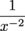is the same as x2. Could life be any more awesome right now?

This brings us to a new rule: whenever like bases are divided, we subtract the exponent in the denominator from the one in the numerator.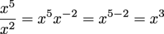This is called the quotient rule.

You might want to think of it this way: Multiplying out the numerator and denominator gives us.

That's five x's on top and just two x's below. Since x divided by x is 1, we can divide out two x's on the top and bottom. This leaves us with three x's, otherwise known as x3.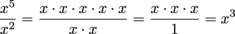The same thing works in the other direction too—if the bigger exponent is in the denominator. This is important in case we get asked to simplify using only positive exponents. And you know that's gonna happen.

### Sample Problem

Simplify using positive exponents: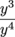.

We told you so. We need to take the lovely exponent in the numerator and subtract it from the exponent in the denominator.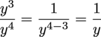### Sample Problem

Simplify: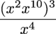.

Yikes, we're going right for the jugular here; all three rules at once. First, we're going to take care of what's in the parentheses by adding exponents.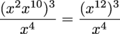Next, we'll multiply 12 by 3 to get 36 before subtracting 4.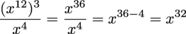As long as we can add, multiply, and subtract, we're golden.

### Sample Problem

Simplify using positive exponents: (-8z -6)(7z3) .

In case you weren't awake in the first section, coefficients are the numbers in front of or multiplied by the variables. In this problem, the -8 and 7 are coefficients. This tends to make things just a bit more confusing because we still need to treat the coefficients like normal numbers while applying exponent rules to the exponents.

In this particular problem, we multiply the -8 and 7 while adding the exponents.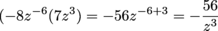Next.

### Sample Problem

Simplify using positive exponents: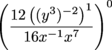.

If you went ahead and did all the work for this one before realizing it was all raised to the power of 0, we apologize. Okay, we don't really apologize. We may or may not be secretly laughing. But remember, anything raised to the 0 is 1. Anyway, here's our work for this problem-o.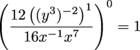We won't trick you with the next one. We actually do promise this time.

### Sample Problem

Simplify using positive exponents: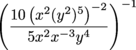.

Whoa. Stepping it up is an understatement. This is crazy-looking, but it's definitely a good summary of all our rules up to this point. We're sure it's no problem for a well-trained Shmooper like you.

All we need to do is keep the x's with the x's and the y's with the y's, and deal with the coefficients separately. To start, we'll take care of the stuff inside the parentheses in both the numerator and denominator. We'll work inside out using the product and power rules.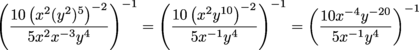We can also take care of those pesky coefficients by dividing 10 by 5.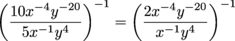Things are already looking up. Next, since we need positive exponents, we can use the quotient rule for the x's and y's separately. Of course, we're still inside the parentheses.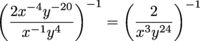Finally, the -1 exponent can be multiplied to both of the other exponents as well as the whole number in the numerator. When you get really good, you'll see that a -1 exponent really just flips the fraction.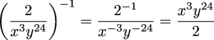This can also be written as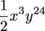. Your call. It's correct either way.